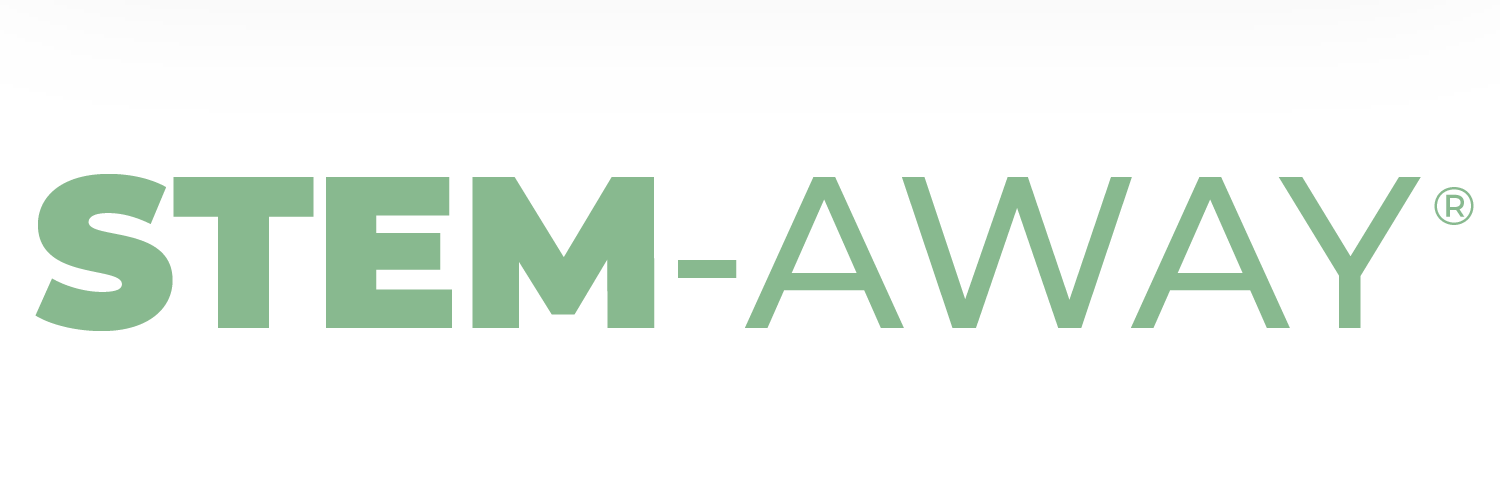# Quality Control and Data Visualisation - Sona Popat

Progress Summary - Quality Control and Data Visualisation - Sona Popat

Presentation:

Contribution: completed the code to generate the plots, created and delivered the presentation

Challenges Faced:

• I had a few minor technical issues with getting used to using R’s syntax and an error with R saying that not enough memory had been allocated for a function to run, but these were overcome using troubleshooting resources such as office hours and the troubleshooting channel.

• My main challenge was with producing the PCA plots - initially the plots produced did not look as expected due to what I thought was an error in the batch correction step. In actual fact, this was due to the code used to colour the PCA plot points, which I initially based on the training materials. Only when I independent tried troubleshooting using the help pages and StackOverflow and learned more about the functions, then trying to vary the code myself, did I manage to successfully colour the PCA plot points. I did this by adding the sample type column from the batch metadata to the data frame being plotted instead of manually grouping the points into cancer or normal categories (code and figures shown below).

Before:

``````##Plot PCA plot - colour by sample type

group_3 <- factor(c(rep("control", 47), rep("cancer", 47)))

pca_plot_3 <- ggplot(df_out_3, aes(x = PC1, y = PC2, color = group_3, label = as.character(c(1:94))))

pca_plot_3 <- pca_plot_3+geom_point()+stat_ellipse()+ xlab(percentage) + ylab(percentage)+ geom_text(aes(label=as.character(c(1:94))),hjust=0, vjust=0, size = 5) + theme(text = element_text(size=20))

pca_plot_3
``````

After:

``````##Plot PCA plot - colour by sample type

pca_plot_3 <- ggplot(df_out_3, aes(x = PC1, y = PC2, color = CN, label = as.character(c(1:94))))

pca_plot_3 <- pca_plot_3+geom_point()+stat_ellipse()+ xlab(percentage_3) + ylab(percentage_3)+ geom_text(aes(label=as.character(c(1:94))),hjust=0, vjust=0, size = 5) + theme(text = element_text(size=20))

pca_plot_3 <- pca_plot_3 + ggtitle("PCA plot of normalised and batch corrected data") + theme(plot.title = element_text(hjust = 0.5)) + scale_colour_discrete("Sample type")

pca_plot_3
``````

We can see that the position of the points in the plot does not change - only the colour changes. This is because the samples were not organised in a list so all of the normal and cancer samples were together; they were mixed up, so the grouping created at the beginning of the “before” code was incorrectly labelling the samples.

Summary of Work:

• Trying quality control using the different methods to see if the same outliers were identified

• Quality control using affyPLM - produced RLE and NUSE boxplots and histograms

• Normalisation using grcma

• Batch correction

• Visualisation using PCA plots

• Organised and led our sub-group meeting

• Wrote minutes for the sub-group meeting and communicated these to members afterwards

• Created and delivered a presentation summarising the week’s progress

Further Notes:

I found it really interesting learning how outliers are identified and data is processed ready for DGE analysis, especially in large datasets. The batch correction was the most interesting step for me, as it shows how data from different sources can be compiled into a larger data set - and this is becoming more and more common as science becomes more collaborative across the world.

I would be interested to know more about how to select which quality control method, as several different options were covered in the pipeline we used. It would be useful to know the advantages and disadvantages of each one, and when it is most appropriate to use each one.